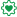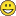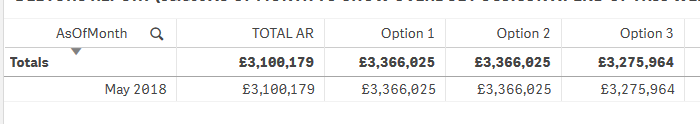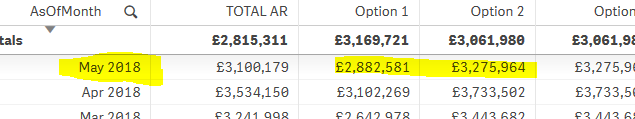# Qlik Design Blog

All about product and Qlik solutions: scripting, data modeling, visual design, extensions, best practices, etc.

Announcements
Coming your way, the Qlik Data Revolution Virtual Summit. October 27-29. REGISTEREmployee

## A Primer on Set Analysis

Set analysis is one of the more complex things you can use in QlikView or Qlik Sense. Its syntax is often perceived as complicated and there are some misunderstandings around it. So here is my short explanation.

Set analysis is a way to define an aggregation scope different from current selection. Think of it as a way to define a conditional aggregation. The condition, however, is in itself like a selection that is evaluated before the cube (the chart) is expanded. Hence, it is not possible to have a condition that is evaluated row by row.

The set analysis is used inside an aggregation function, e.g. in an expression like

Sum(Sales)

The first step is to add the markers for the set analysis – the curly brackets:

Sum( {…} Sales)

These define the record set over which the aggregation should be made. Inside, you can use different identifiers and operators, e.g. ‘\$’ for records implied by current selection, ‘1’ for all records, ‘1-\$’ for all excluded records, etc.

A set of records that you can define by a simple selection is called natural set. Not all record sets are natural; for instance, {1-\$} cannot always be defined through a selection.

The next step is often to add a set modifier, which is defined by angle brackets. The set modifier adds or changes a selection. It can be used on any natural set and consists of a list of fields, where each field can have a new selection:

Sum( {\$<…>} Sales)

The next step is to define the element set for a field; the set of field values that defines the selection. The element set could be a field reference or a set function, P() or E(). It is more common, though, that it is an explicit list of field values or a search, and then you need the curly brackets to define the element set:

Sum( {\$<Date={…}>} Sales)   or   Sum( {\$<Date=P(…)>} Sales)

A search can be defined through double quotes. This way, field values that match the search string will be selected:

Sum( {\$<Date={"…"}>} Sales)

Do not use single quotes to initiate a search here! Single quotes should denote literals, i.e. explicit field values. (Yes, today single quotes work as a search, but this is a bug that one day will be fixed…)

Often a numeric search is made, defined by a leading relational operator. Then, field values will be selected based on a comparison:

Sum( {\$<Date={"<=…"}>} Sales)

To make it worse, the value to which the field values are compared is often a calculated one. And in order to get a calculated value into the expression, a dollar expansion is needed.:

Sum( {\$<Date={"<=\$(…)"}>} Sales)

Inside the dollar expansion, you need an expression that starts with an equals sign and contains an aggregation function, e.g.:

Sum( {\$<Date={"<=\$(=Max(Date))"}>} Sales)

This aggregation function is evaluated globally, before the cube is expanded.

As you can see, there are many levels of a set expression, and many pairs of brackets and delimiters that need to match. When you write set expressions, you should always write both brackets directly, and then continue with the expression between them. This way you will avoid simple syntax errors.

Good luck with your set analysis expressions!

HIC

Further reading related to this topic:

Why is it called Set Analysis?

Dates in Set Analysis

Excluding values in Set Analysis

Introduction to Set Analysis (video) - Part 1Creator II

Great article! It got me answers to a number of my current issues.

Thanks12,289 ViewsCreator

Hi Henric,

I'm interested in your tips on Sum( {\$<Date={"<=\$(=Max(Date))"}>} Sales)

you mentioned there the function in the element area is evaluated globally.

Is there any way to make the function work under the dimension in straight tables?

Thanks.

Actually I asked this for the latest discussion I start in the blog

https://community.qlik.com/message/1130102#1130102

Thanks in advance.Best Regards.

12,289 ViewsEmployee

I assume you mean that the function should be evaluated once per dimensional value. If so, the answer is "No". Set analysis is like a selection that is made before the chart is calculated. So it is not possible to write a set analysis expression that is evaluated to different values within the same chart.

HIC

12,289 ViewsNot applicable

Thanks for this wonderful explanation Henric! I was just performing was fact table concatenation and was looking for an explanation on how to handle the different data.

12,289 ViewsCreator

Thanks for Sharing

12,289 ViewsSpecialist III

Hi Henric (or anyone who can answer this)

Would it be possible to make this possible in set analysis. To have something like P or E (say D) where set analysis can be linked to the dimension?

I have a situation with debtors where there are a lot of credits that i want to take out. I can easily do this with the closing debtor position (or any Month). Like so

sum({\$<CustAccNum = {"=sum(ARValue) >0"}>}ARValue)

where I select one AsOfMonth

But it doesn't work with AsOfMonth as a dimension.

So with only one AOM selected the total are correct (option 1 and 2)

Option 1 >>>  sum({\$<CustAccNum = {"=sum(ARValue) >0"}>}ARValue)

Option 2 >>>> sum({\$<CustAccNum = {"=sum(if(AsOfMonth = AsOfMonth ,ARValue)) >0"}>}ARValue)

Option 3 >>>   sum({\$<CustAccNum = {"=sum({1}if(AsOfMonth = AsOfMonth ,ARValue)) >0"}>}ARValue)But when the AsOfMonth May 2018 selection is excluded (the correct figure is £3,366,025). So option 2 (and 3) is close but there is always a smallish differenceI tried everything I could think of to get the correct figure as above. Including various options in script. Maybe there is some way to do this but it would be so easy if the set analysis could be linked to the dimension

Something like this

sum({\$<CustAccNum = {"=sum({\$<AsOfMonth = D(AsOfMonth) >} ARValue)>0"}>}ARValue)

Thanks.

12,289 Views